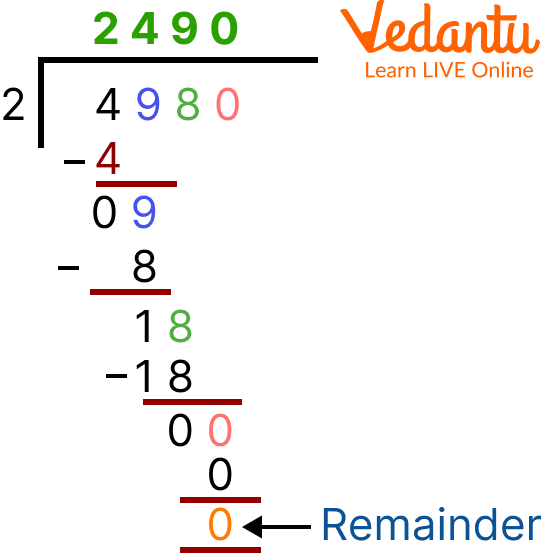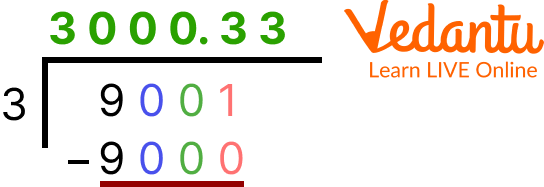Courses
Courses for Kids
Free study material
Free LIVE classes
More

# 4 Digit DivisionLIVE
Join Vedantu’s FREE Mastercalss

## How to Solve 4 Digit Division Sums?

Today, we will be learning 4-digit divisionsums in the easiest way possible. As we all know that division is one of the basic arithmetic operations where we get a quotient (result) when we divide the dividend with the divisor and if not a whole number, then a remainder.

We use division in cases where we want to divide the quantities or numbers equally or into equal sets and especially in finding out the HCF of given numbers.

An obelus symbol (÷ or /) is used to represent the mathematical operation of division. It is another mathematical operation we use in Arithmetic problems.

## Elements of Division

Here's a one-by-one element of long 4-digit division sums with the following example.

• Dividend: The dividend is the number you are dividing up with

• Divisor: It is the number that is supposed to divide the dividend.

• Quotient: It is the final result that we obtain after dividing.

• Remainder: If the answer to the division problem is not a whole number; the rest of the number is called the remainder.Elements of Division

## Important Points to Remember for Division?

If you have an idea of the other 3 mathematical operations listed below and a basic knowledge of divisibility you can master division too!

## Basic Rules to Remember

1. Any number divided by 0 is infinite ⇒ 1 ÷ 0 = infinite

2. 0 divided by any number is 0 ⇒ 0 ÷ 1 = 0

## Division Process

There is a particular formation according to which allot the numbers to their respective places and that is basically called the placement of the elements. Once we put the numbers in their places next we need to follow a few steps to complete the division method and they are:-

Step 1 - Take the first number of the tip from the left-hand side. Check if this number is lesser than or equal to the divisor.

Step 2 - Also divide it by the divisor and write the answer on top as the quotient.

Step 3 - Abate the result from the number and write the difference below.

Step 4 - Bring down the coming number of the tip( if present).

Step 5 - Repeat the same process until it's non-divisible or you get 0 as the remainder.

Let’s try understanding it with a few examples of how to solve division sums

1. 4980 ÷ 2 =?

We need to divide the number 4980 by 2 which means 2 is the divisor and 4980 is the dividend.

The number in the thousands place value which is 4 is greater than 2 so, no need to take two digits at a time and 4 is a multiple of 2 as well so, therefore, we take the number directly and 0 is put in the quotient's place.Example 1 of Division Steps

4980 ÷ 2 = 2490

2. 9001÷3 =?

The divisor is 3 and the dividend is 9001; so, we will place them according to their places.

Note: We put 0 in the quotient when a number is completely divided and the remainder that we get is 0. Putting the 0 simultaneously in the quotient’s place as well; So basically considering 9000 (round of 9001) as a multiple of 3. For better understanding follow the image shown below. Putting point ( . ) in the quotient’s place means we can add 0 as the one place to the dividend.Example 2 of Division Steps

If the quotient goes into decimals, then it is better to consider only two numbers after the point or round of those two numbers also works.

Ans: 9001 ÷ 3 = 3000.33

3. \$\dfrac{2272}{4}\$

The divisor is 4 and the dividend is 2272; so, we will place them according to their places.

\$\dfrac{2272}{4} = 568\$

The remainder will be zero and the quotient is 568.

4. \$\dfrac{7128}{9}\$

The divisor is 9 and the dividend is 7128; so, we will place them according to their places.

\$\dfrac{7128}{9} = 792\$7128/9 = 792

The remainder will be 0, the quotient is 792.

5. \$\dfrac{2335}{5}\$

The divisor is 5 and the dividend is 2335; so, we will place them according to their places.

\$\dfrac{2335}{5} = 467\$

The remainder is 0, the quotient is 467.

## Practice Questions

How do solve these four-digit division sums?

Q 1. \$\dfrac{5090}{4}\$

Ans:1272.5

Q 2. \$\dfrac{7080}{12}\$

Ans: 590

Q 3. \$\dfrac{2103}{9}\$

Ans:233.67

Q 4. \$\dfrac{4312}{5}\$

Ans: 862.4

Q 5. \$\dfrac{7094}{7}\$

Ans: 1013.42

## Summary

In this article, proper steps of the 4-digit number long division method are shown with the use of a few examples for better understanding. The division is a basic mathematical operation where a larger number is split into smaller numbers containing equal groups. Basic rules of division have also been explained along with the division steps.

It is a very useful method with its own application as per the need. A vast number of examples are available to practice the 4-digit division sums and anyone can easily solve them. We will come up with more easy methods to solve mathematical operations and problems so keep visiting our blog for more updates.

Last updated date: 20th Sep 2023
Total views: 109.8k
Views today: 1.09k

## FAQs on 4 Digit Division

1. What are the properties of division when Zero is either the dividend or divisor?

There are two properties of division that apply to the number 0. One applies when 0 is the dividend. The reason for the same is that you can’t make parts of something that have no parts or values to divide into. The second property mentions what happens when 0 is the divisor. When a nonzero number is divided by 0 the answer is undefined. The reason for the same is that if 0 is multiplied by any number the product is always 0. Thus, 0 can't be multiplied by any nonzero number to give the answer as the dividend.

2. What is the importance of division?

The division allows us to divide or 'share' numbers to find an answer. For example, let's consider how we would find the answer to 10 ÷ 2 (ten divided by two). This is the same as 'sharing' 10 sweets between 2 children.

3. How division is used in real life?

Suppose a girl buys a bag that has 340 pieces of candy in it. She wants to divide the candy into treat bags for her students for an upcoming holiday. How many pieces should each child get? Now, obviously, a teacher is going to give equal numbers, so the student has to divide. In this way, division can be implemented in real life.